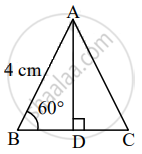# Find the height of an equilateral triangle having side 4 cm? - Geometry

Sum

Find the height of an equilateral triangle having side 4 cm?

#### SolutionLet ∆ABC be the given equilateral triangle.

∴ ∠B = 60°      ......[Angle of an equilateral triangle]

Let AD ⊥ BC, B – D – C.

In ∆ABD, ∠B = 60°, ∠ADB = 90°

∴ ∠BAD = 30°      ......[Remaining angle of a triangle]

∴ ∆ABD is a 30° – 60° – 90° triangle.

∴ AD = sqrt(3)/2 AB    ......[Side opposite to 60°]

= sqrt(3)/2 xx 4

= 2sqrt(3) units

∴ The height of the equilateral triangle is 2sqrt(3) units.

Concept: Application of Pythagoras Theorem in Acute Angle and Obtuse Angle
Is there an error in this question or solution?

Share